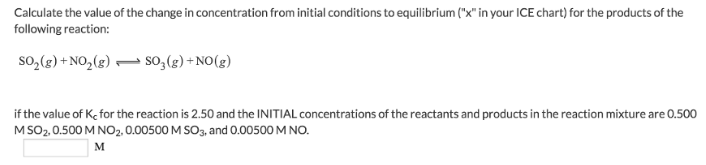# Problem: Calculate the value of the change in concentration from initial conditions to equilibrium ("x" in your ICE chart) for the products of the faollowing reaction:SO2(g) + NO2(g) ⇌ SO3(g) + NO(g)If the value of Kc for the reaction is 2.50 and the INITIAL concentrations of the reactants and products in the reaction mixture are 0.500 M SO2, 0.500 M NO2, 0.00500 M SO3, and 0.00500 M NO.

###### FREE Expert Solution
79% (418 ratings)###### Problem Details

Calculate the value of the change in concentration from initial conditions to equilibrium ("x" in your ICE chart) for the products of the faollowing reaction:

SO2(g) + NO2(g) ⇌ SO3(g) + NO(g)

If the value of Kc for the reaction is 2.50 and the INITIAL concentrations of the reactants and products in the reaction mixture are 0.500 M SO2, 0.500 M NO2, 0.00500 M SO3, and 0.00500 M NO.